# NCERT Solutions For Class 10 Maths Chapter 7 Coordinate Geometry Ex 7.1

Get Free NCERT Solutions for Class 10 Maths Chapter 7 Ex 7.1 Coordinate Geometry Class 10 Maths NCERT Solutions are extremely helpful while doing homework. Exercise 7.1 Class 10 Maths NCERT Solutions were prepared by Experienced ncert-books.in Teachers. Detailed answers of all the questions in Chapter 7 Maths Class 10 Coordinate Geometry Exercise 7.1 Provided in NCERT Textbook

## NCERT Solutions For Class 10 Maths Chapter 7 Coordinate Geometry Ex 7.1

NCERT Solutions for Class 10 Maths Chapter 7 Coordinate Geometry Ex 7.1 are part of NCERT Solutions for Class 10 Maths. Here we have given NCERT Solutions for Class 10 Maths Chapter 7 Coordinate Geometry Ex 7.1.

Question 1.
Find the distance between the following pairs of points:
(i) (2, 3), (4, 1)
(ii) (-5, 7), (-1, 3)
(iii) (a, b), (-a, -b)
Solution: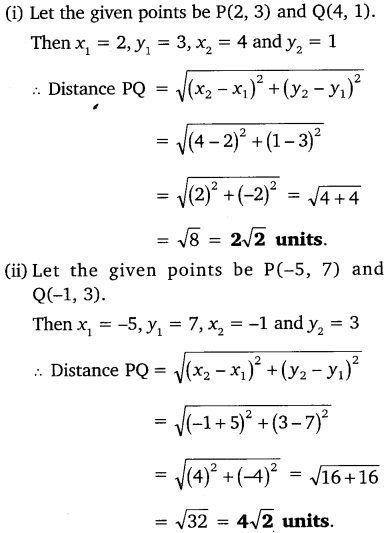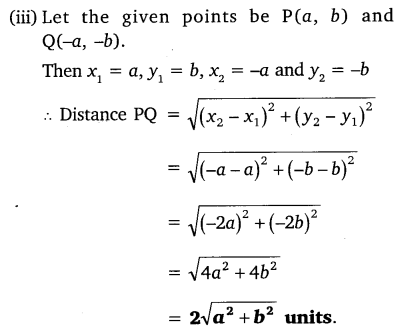Question 2.
Find the distance between the points (0, 0) and (36, 15).
Solution: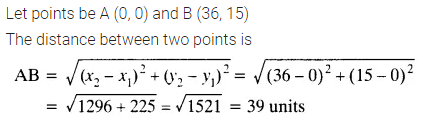You can also download the free PDF of Chapter 7 Ex 7.1 Coordinate Geometry NCERT Solutions or save the solution images and take the print out to keep it handy for your exam preparation.

Question 3.
Determine if the points (1, 5), (2, 3) and (-2, -11) are collinear.
Solution:Question 4.
Check whether (5, -2), (6, 4) and (7, -2) are the vertices of an isosceles triangle.
Solution:Question 5.
In a classroom, 4 friends are seated at the points A, B, C and D as shown in the given figure. Champa and Chameli walk into the class and after observing for a few minutes Champa asks Chameli, “Don’t you think ABCD is a square?” Chameli disagrees. Using distance formula, find which of them is correct.Solution:Question 6.
Name the type of quadrilateral formed, if any, by the following points, and give reasons for your answer.
(i) (-1, -2), (1, 0), (-1, 2), (-3, 0)
(ii) (-3, 5), (3, 1), (0, 3), (-1, -4)
(iii) (4, 5), (7, 6), (4, 3), (1, 2)
Solution: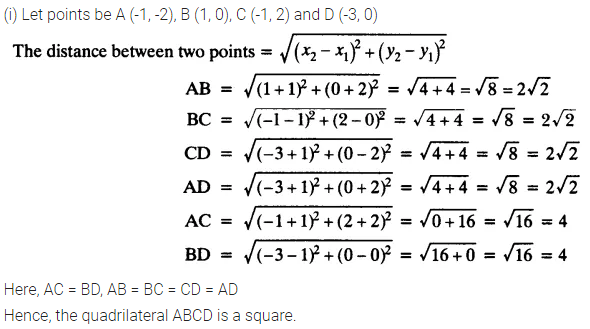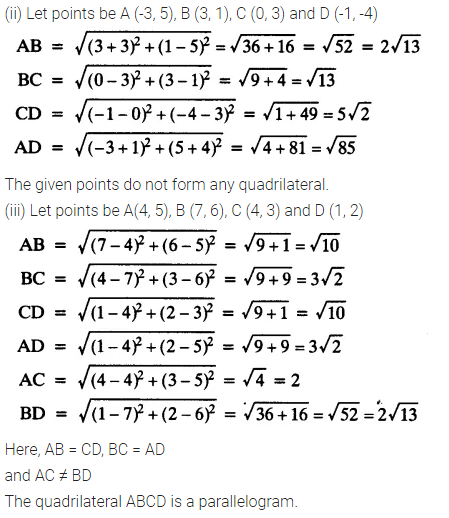Question 7.
Find the point on the x-axis which is equidistant from (2, -5) and (-2, 9).
Solution: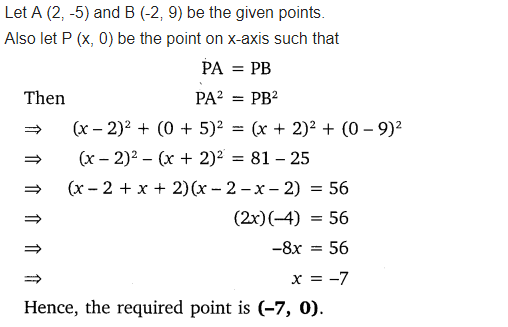Question 8.
Find the values of y for which the distance between the points P (2, -3) and Q (10, y) is 10 units.
Solution: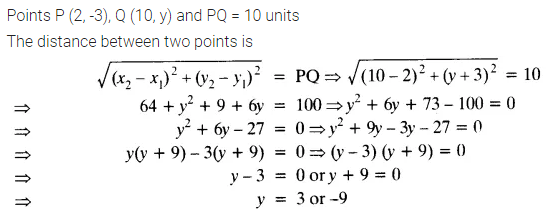Question 9.
If Q (0, 1) is equidistant from P (5, -3), and R (x, 6), find the values of x. Also, find the distances QR and PR.
Solution: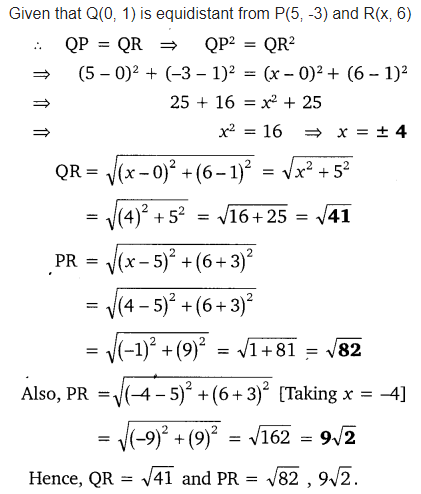Question 10.
Find a relation between x and y such that the point (x, y) is equidistant from the points (3, 6) and (-3, 4).
Solution:error: Content is protected !!
+